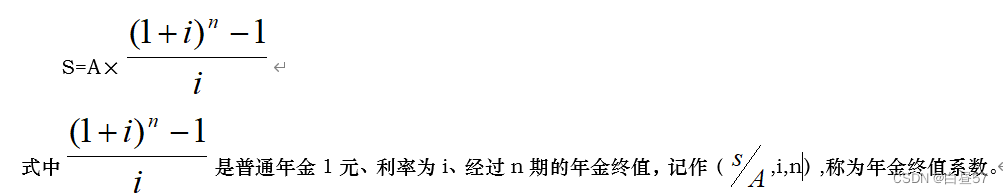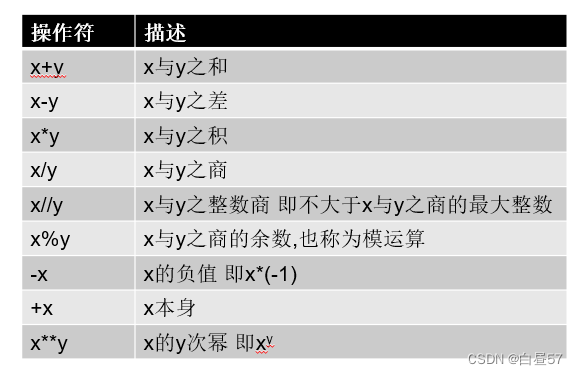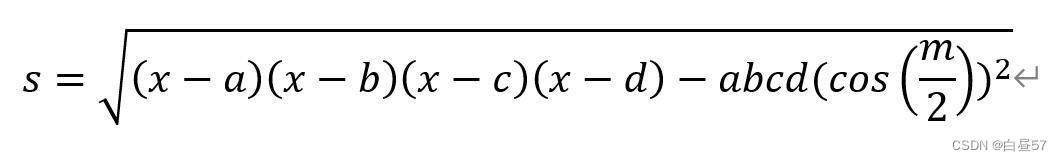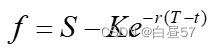# 实验一 数字类型及其操作（Python，educoder）

### 任务描述### 测试说明

``````#计算普通年金终值
A=eval(input("请输入每年本金"))
i=eval(input("请输入利率"))
n=eval(input("请输入年限"))
#代码开始
F=A*(((1+i)**n-1)/i)
#代码结束
print("普通年金终值是{:.2f}".format(F))
``````

### 相关知识### 测试说明

``````import math
a=eval(input(""))
b=eval(input(""))
c=eval(input(""))
#代码开始
p=a+b+c
s=(a+b+c)/2
S=math.sqrt(s * (s - a) * (s - b) * (s - c))
print("三角形周长{:.2f}三角形面积{:.2f}".format(p,S))
#代码结束``````

### 任务描述### 相关知识

math库的函数

math库包括16个三角曲线函数 sin(x) 返回x的正弦函数值 cos(x)返回x的余弦函数值 tan(x) 返回x的正切函数值 radians(x) 角度x的角度值转弧度值 degree(x) 角度x的弧度值转角度值 注意：在这些三角函数中，要求x是弧度 相关课件和视频 math库

### 测试说明

``````import math
a=eval(input("请输入边长一"))
b=eval(input("请输入边长二"))
c=eval(input("请输入边长三"))
d=eval(input("请输入边长四"))
m=eval(input("请输入对角之和"))
#代码开始
z=a+b+c+d
x=(a+b+c+d)/2
#代码结束
print("四边形周长{:.2f}面积{:.2f}".format(z,s))

``````

### 相关知识

for 循环变量 in range(初值,终值，步长)： <循环体> 从初值到终值（不包含终值）逐一提取元素（每次增加步长值，步长值默认为1），放在循环变量中，对于所提取的每个元素执行一次循环体 例如 对1到10求和 s=0 for i in range(1,11,1): s=s+i print(s)

1. `x=56`
2. `x1=x%10（个位）`
3. `x2=x//10(十位)`
4. `print("各数位之和为",x1+x2)`

### 测试说明

``````#代码开始
for i in range(100,1000):
a = i//100
b = (i-a*100)//10
c = (i-a*100-b*10)
if i == pow(a,3)+pow(b,3)+pow(c,3):
print(i)
#代码结束

``````

### 相关知识

for循环

for i in range(初值,终值,步长): <语句块>
i从初值变化到终值（不包含到终值），每次增加步长值，对于每个i值执行一次程序块 初值默认为0，步长值默认为1 例如，计算1到100的和s1

1. `s1=0`
2. `for i in range (1,101):`
3. `s1=s1+i`
4. `print(s1)`

1. `s=0`
2. `for i in range (2,101,2):`
3. `s2=s2+i`
4. `print(s)`

### 编程要求### 测试说明

``````#代码开始
x=500000
y=0
for i in range(3,33,3):
for j in range(3):
y=y+y*0.0175+12000
x=x+x*0.04*3
print("第{:2}年公寓方案{:.2f}大额存单投资收益{:.2f}".format(i,y,x-500000))
#代码结束

``````

### 相关知识

for循环

for i in range(初值,终值,步长): <循环体>

### 测试说明

``````#年度总和法固定资产折旧
x=eval(input("固定资产原始价值"))
y=eval(input("预计净残值"))
n=eval(input("使用年限"))
#代码开始
z=x
for i in range(0,n):
s=(x-y)*(n-i)/(n*(n+1)/2)
z=z-s
print("第{}年折旧额{:.2f}剩余价值{:.2f}".format(i+1,s,z))
#代码结束``````

### 任务描述S为资产现货价格，K为远期合约的交割价格，r为无风险年利率，T为远期合约的到货时间，t是现在的时间，T-t代表远期合约距离到期时间的剩余时间，均以年为单位，

### 测试说明

``````import math
s=eval(input("现货价格"))
k=eval(input("远期价格"))
r=eval(input("利率"))
t=eval(input("剩余时间"))
#代码开始
f=s-k*math.exp((-r)*t/12)
#代码结束
print("远期价值{:.2f}".format(f))``````

### 测试说明

``````#开始
n = int(input(""))

if n == 1:
print("100.000000")
else:
i = 0
s = 100
while i < (n-1):
s = s + 100/(2**i)
i += 1
print("{:.6f}".format(s))
#结束
``````